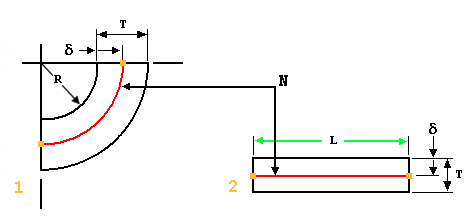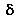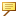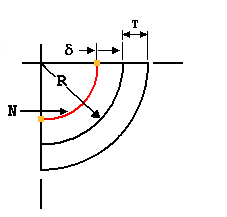About Y Factor and K Factor
Y factor and K factor represent part constants used in formulas to calculate the developed length of flat material required to make a bend of a specific radius and angle in a design. Y factor and K factor are defined by the location of the material's neutral bend line with respect to the thickness. The neutral bend line’s position varies according to the type of material used for a board. The numeric references range from 0 to 1. The numeric references for Y factor and K factor can be negative, with the lower numbers representing softer material. The length of the neutral bend line is equal to the developed length.
K factor is a ratio between the distance from the neutral bend line to the inside bend radius and the material thickness. K factor uses the formula K factor = δ/T. Y factor uses the formula Y factor = K factor * (Π/2). The default value for Y factor is 0.50.
Developed Length of Material and Y Factor and K Factor1. Bent condition
2. Flat Condition
Where:= Distance between the inside radius of the bend and the neutral bend line
T = Material thickness
L = Length of neutral bend lineFor stretched bends δ is negative. As a result, the neutral layer stays out of the material thickness causing the Y factor and K factor to be negative.